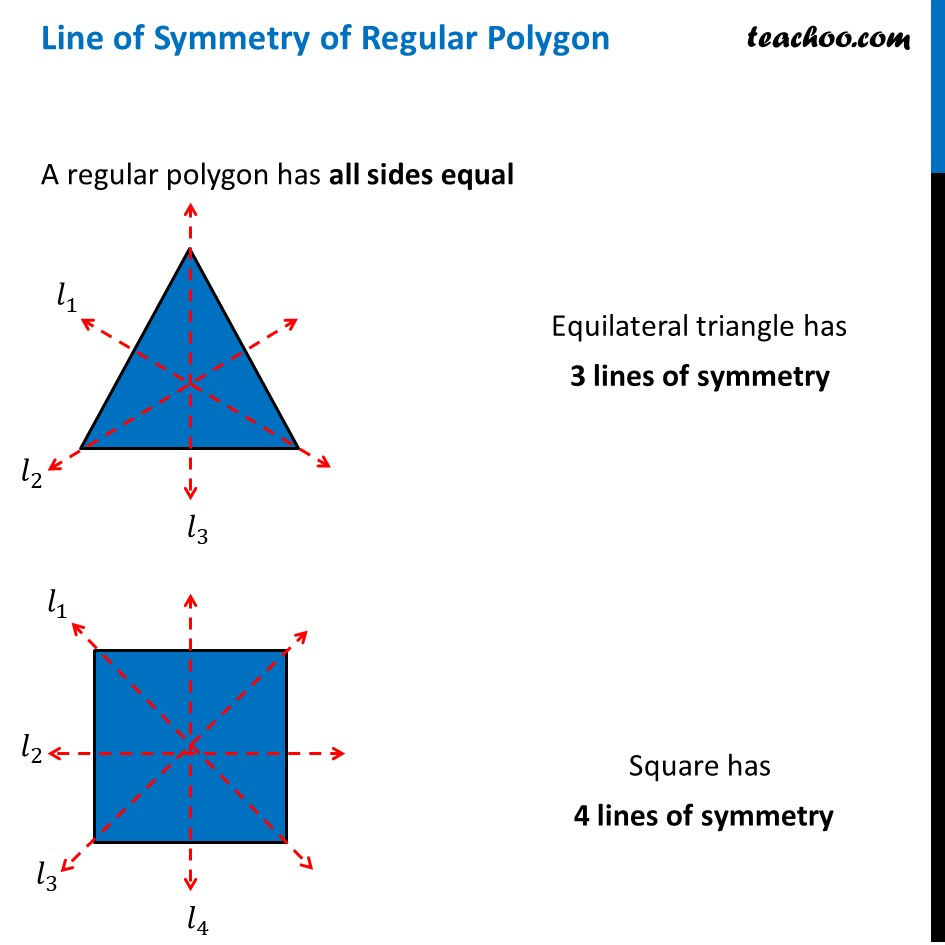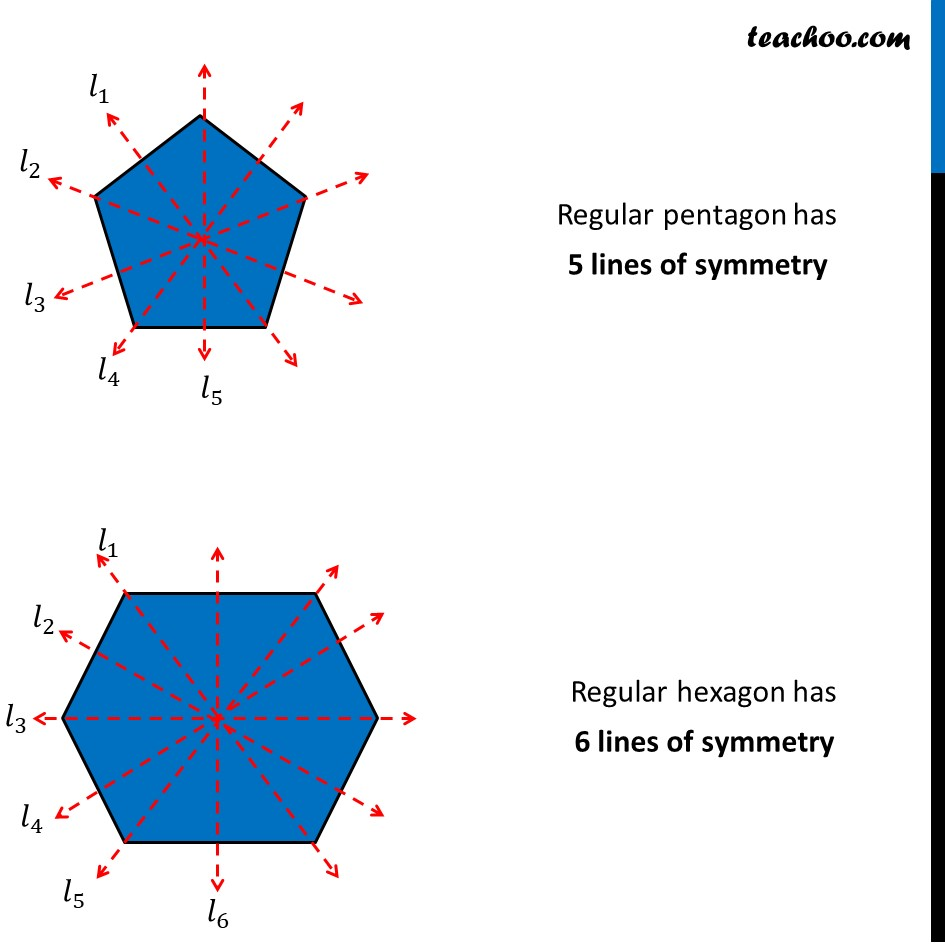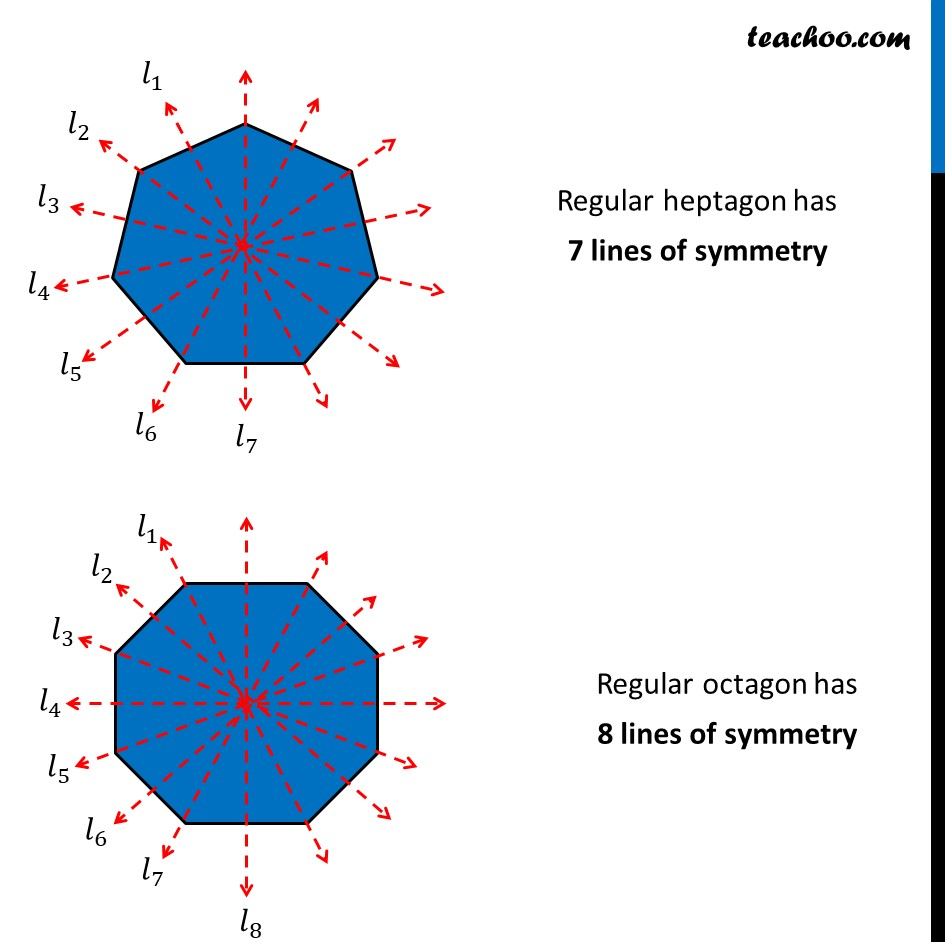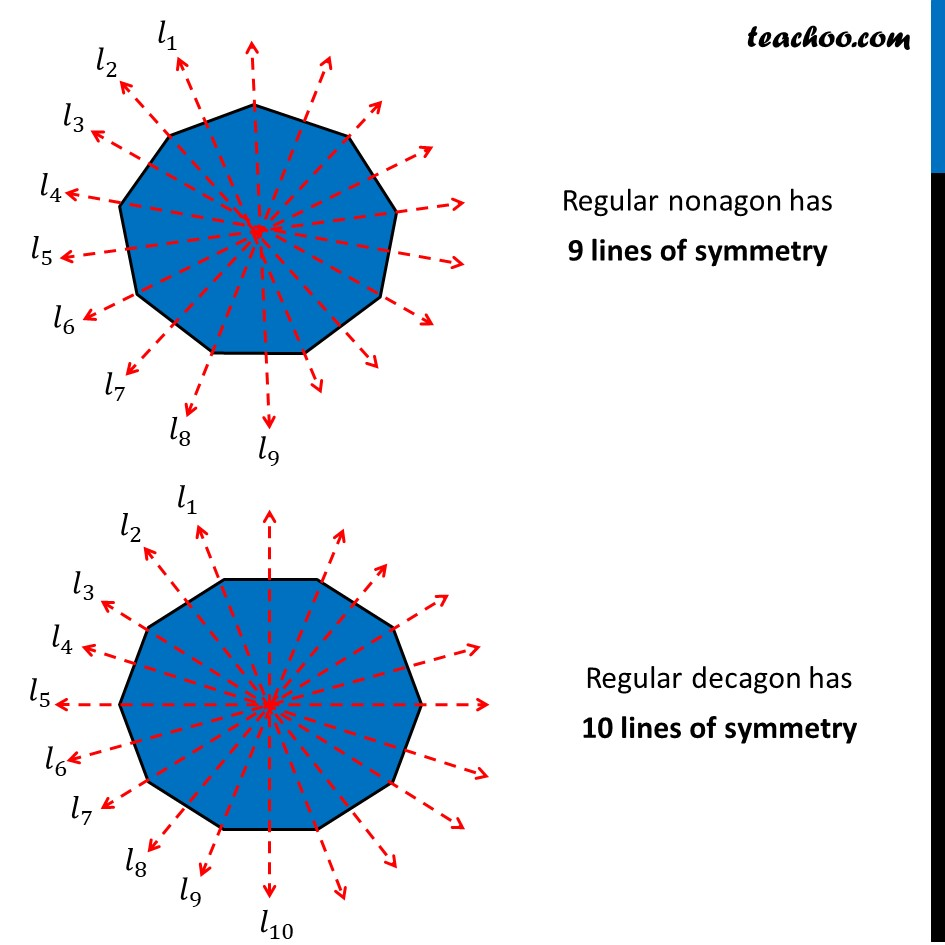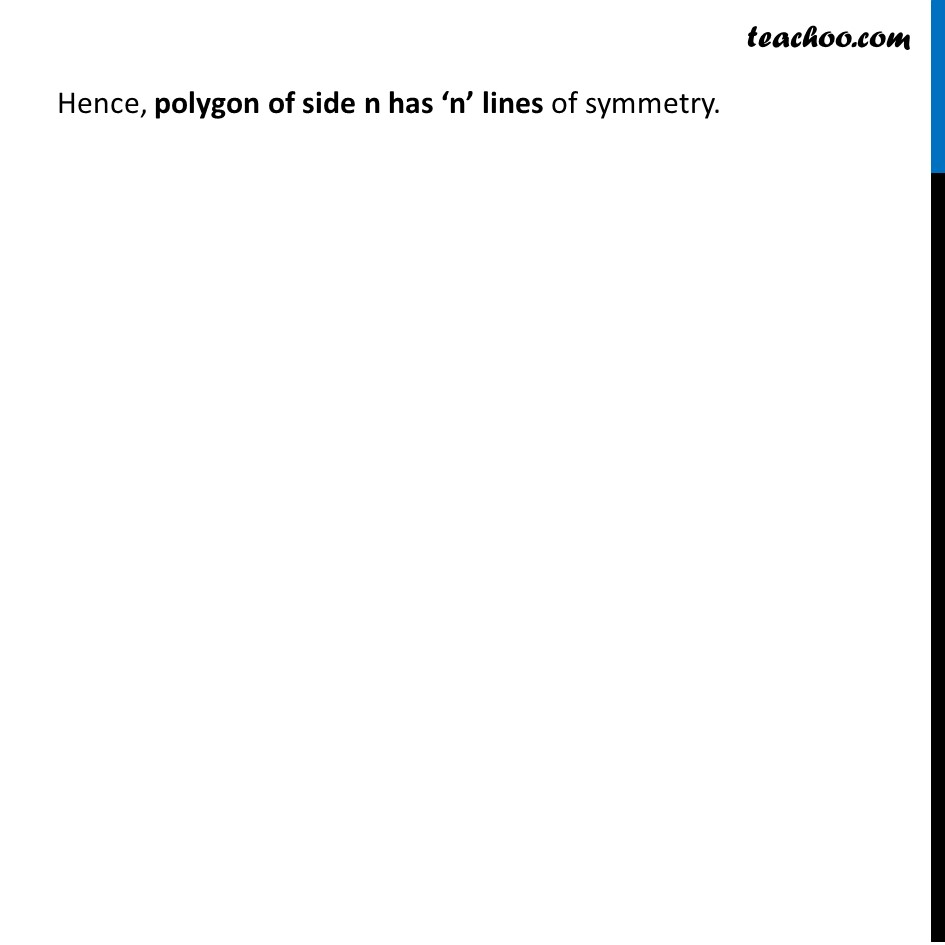1. Chapter 13 Class 6 Symmetry
2. Concept wise
3. Figures with more than one lines of symmetry

Transcript

Line of Symmetry of Regular Polygon A regular polygon has all sides equal Equilateral triangle has 3 lines of symmetry Square has 4 lines of symmetry Regular pentagon has 5 lines of symmetry Regular hexagon has 6 lines of symmetry Regular heptagon has 7 lines of symmetry Regular octagon has 8 lines of symmetry Regular nonagon has 9 lines of symmetry Regular decagon has 10 lines of symmetry Hence, polygon of side n has ‘n’ lines of symmetry.

Figures with more than one lines of symmetry

Chapter 13 Class 6 Symmetry
Concept wise

About the AuthorDavneet Singh
Davneet Singh is a graduate from Indian Institute of Technology, Kanpur. He has been teaching from the past 10 years. He provides courses for Maths and Science at Teachoo.